Create a hot spot map

If you needed money to consolidate debt, pay for a wedding, take a vacation, fix your home, or cover unexpected bills, would you apply for an online loan? Over the past several years, millions of people have answered with a yes. If you choose to join them, what will your interest rate be? Most people take for granted that a poor credit score translates to a higher interest rate. Is that a valid assumption?

Jonathan Blum, a New York-based author and GIS novice, wants to know more. Using loan data from August 2007 to September 2015 in the United States (acquired from LendingClub and summarized by 3-digit ZIP Code area), he intends to confirm whether the average interest rates people pay for their online loans vary geographically.

First, you'll create a hot spot map that shows areas with statistically significant high or low interest rates.

Open the project

You'll download and open an ArcGIS Pro project containing loan data summarized by 3-digit ZIP Code areas.

2. Right-click the downloaded folder and extract it to a location you can easily find, such as your Documents folder.
3. Open the online-lending-data folder.

The folder contains a file geodatabase with data, an index folder, an ArcGIS Pro project file, and an ArcGIS toolbox.

4. If you have ArcGIS Pro installed on your machine, double-click the OnlineLending project file (it may have an .aprx extension). If prompted, sign in using your licensed ArcGIS account.
Note:

If you don't have ArcGIS Pro or an ArcGIS account, you can sign up for an ArcGIS free trial.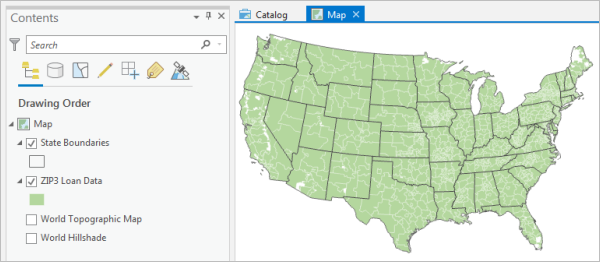The project contains a map of the continental United States. It has a layer of state outlines and a layer of ZIP3 areas with loan data. (ZIP3 areas are the geometry defined by the first three digits of a standard 5-digit ZIP Code).

You'll open the loan data's attribute table to familiarize yourself with its data.

5. In the Contents pane, right-click ZIP3 Loan Data and choose Attribute Table.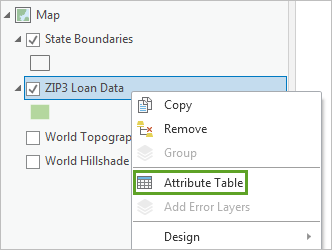The table opens. For each ZIP3 area there is a ZIP3 identifier, the total number of loan applications submitted, the total number of loans issued (accepted loans), the average interest rate for all loans issued, the average loan grade ranking for all loans issued, and the total number of households.

LendingClub assigns a loan grade to every loan application it receives, ranging from A1 (lowest interest rate) to E5 (highest interest rate). These loan grades were converted to simple numeric rankings for analysis. A1 loan grades were assigned a rank of 1, A2 loan grades were assigned a rank of 2, and so on. The higher the ranking, the riskier the loan tends to be.

6. Close the table.

Select tracts with at least 30 loans

To ensure the average interest rate reported for each ZIP3 area is both reliable and representative, you'll focus your analysis on ZIP3 areas where at least 30 loans have been funded. First, you'll run the Select Layer By Attribute geoprocessing tool to select all ZIP3 areas with 30 or more issued loans.

1. On the ribbon, click the Analysis tab. In the Geoprocessing group, click Tools.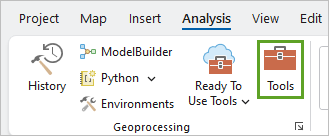The Geoprocessing pane appears. This pane contains a large number of tools that can be used on data layers.

2. In the Geoprocessing pane, search for and select Select Layer by Attribute.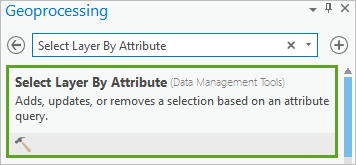The tool opens. You can set several parameters to change how it runs. First, you'll choose which table to run the tool on.

3. For Input Rows, choose ZIP3 Loan Data.

Next, you'll create a clause so that ZIP3 areas with 30 or more loans are selected.

4. For Expression, click New expression.
5. Build the expression Where Number of loans issued is greater than or equal to 30.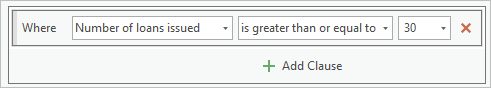6. Click Run.

ZIP3 areas with 30 or more loans issued are selected. Next, you'll create a copy of the layer that contains only the selected ZIP3 areas. You'll be able to use the copied layer for later analysis.

7. In the Geoprocessing pane, click the Back button.8. Clear the existing search text. Search for and open the Copy Features tool.
9. For Input Features, choose ZIP3 Loan Data. For Output Feature Class, keep the default file path and change the output name to ZIP3_Analysis_Data.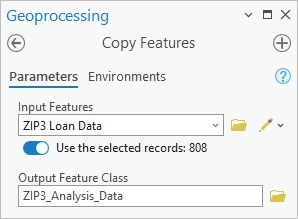When running this tool, if any of the input features are selected, only the selected features will be copied. If no features are selected, all features will be copied.

Note:

By default, output layers are created as a geodatabase feature class. This format is generally superior to the shapefile format, as shapefile attribute field names may be truncated and certain functionality is not supported for them.

10. Click Run.

The ZIP3_Analysis_Data layer is added to the Contents pane. You'll use it for your remaining analyses. You no longer need the ZIP3 Loan Data layer, so you'll remove it from the map.

11. In the Contents pane, right-click the ZIP3 Loan Data layer and choose Remove.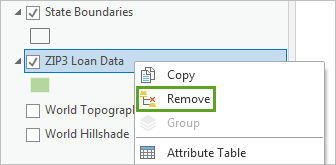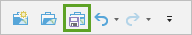Analyze interest rate hot spots

To create a hot spot map of the average loan interest rates, you'll use the Hot Spot Analysis tool. This tool identifies statistically significant clusters of high values and low values.

1. In the Geoprocessing pane, search for and open the Hot Spot Analysis tool.
2. For Input Feature Class, choose ZIP3_Analysis_Data. For Input Field, choose Average Interest Rate.
3. For Output Feature Class, change the output name to Interest_Rate_Hot_Spots.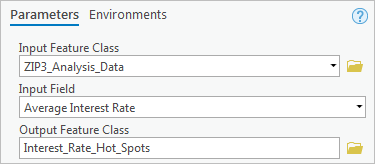The Hot Spot Analysis tool analyzes the statistical significance of each feature value (in this case, each ZIP3 area's average interest rate) within the context of its neighboring features. The Conceptualization of Spatial Relationships parameter defines which features are considered neighbors.

The ZIP3 areas have widely different sizes. Those in the western United States are generally much bigger than those in the east. As such, defining a neighboring feature as a feature that is adjacent to another will cause the scale of the analysis to be inconsistent across the country, skewing your results.

The default option for this parameter, Fixed distance band, defines a neighboring feature by its distance from the feature being analyzed. The advantage of this parameter is that it keeps the scale of your analysis consistent across the study area, ensuring more accurate results.

4. For the Conceptualization of Spatial Relationships parameter, confirm that Fixed distance band is chosen.

You can also specify the distance at which features are considered neighboring. If no distance is set, the tool uses the minimum distance to ensure every feature has at least one neighbor. Sometimes, this setting causes some features to have only one neighbor and some to have thousands, which often is not the best choice.

For this analysis, individual loan records have already been aggregated into 3-digit ZIP Code areas, so using the minimum valid analysis distance is appropriate.

5. Leave the Distance Band or Threshold Distance parameter empty.

Next, you'll apply False Discovery Rate correction, which adjusts results to account for multiple testing and spatial dependence.

6. Check Apply False Discovery Rate (FDR) Correction.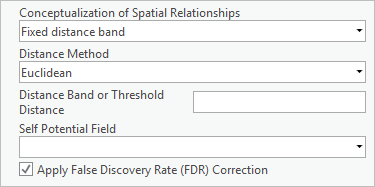7. Click Run.

The tool runs. It computes the average interest rate for each ZIP3 area and all neighboring ZIP3 areas. If this local average interest rate is significantly higher than the average interest rate for all ZIP3 areas across the country, the ZIP3 area being analyzed is designated a hot spot. If it is significantly lower, the ZIP3 area is designated a cold spot. When the tool finishes, a new layer is added to the map.

Note: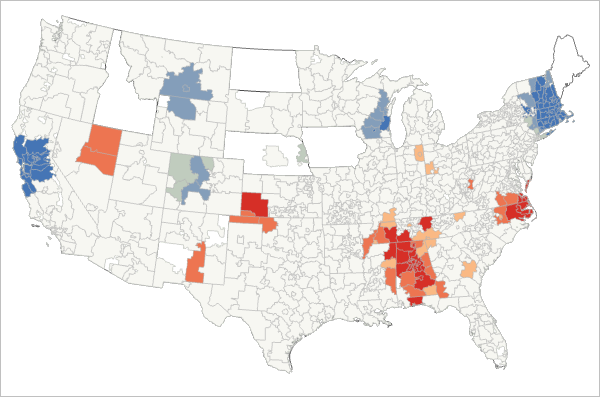The red areas on the map are hot spots, while the blue areas are cold spots. Much of Alabama has higher-than-expected average interest rates, while the area around San Francisco has lower-than-expected interest rates.

8. Save the project.

You've created a hot spot map of average interest rates for ZIP3 areas with a minimum of 30 loans. The map you created shows areas with statistically significant clustering of both high and low average interest rates.

Assessing the hot spot map, Jonathan Blum wonders why interest rates in Alabama are higher than interest rates around San Francisco. Is it fair to assume that the loan grades assigned in Alabama reflect riskier loans? A risky borrower in San Francisco should be just as risky in Alabama, right? Ever the skeptic, Jonathan decides to dig deeper.

Next, you'll dig deeper with him and model the relationship between average interest rates and average loan grades.

Create a regression model

Previously, you created a hot spot map of average interest rate values to see clusters of high and low average interest rates. Next, you'll create a regression model using Generalized Linear Regression (GLR) to determine how well average loan grade rankings predict average interest rates.

A regression model computes the relationship among variables. If average loan grade values effectively predict average interest rate values, your regression model will have a high R-squared value. Additionally, any differences between the model's predictions and observed values (known as residuals) will exhibit a spatially random pattern.

Perform regression analysis

To create a regression model, you'll run the Generalized Linear Regression tool.

1. If necessary, open your OnlineLending project.
2. In the Geoprocessing pane, search and open the Generalized Linear Regression (GLR) (Spatial Statistics Tools) tool.
3. For Input Features, choose ZIP3_Analysis_Data.

A regression model must have a single dependent variable (the variable you want to explain) and one or more explanatory variables. Your dependent variable will be average interest rate.

4. For Dependent Variable, choose Average Interest Rate. For Explanatory Variable(s), check Average Loan Grade Rank.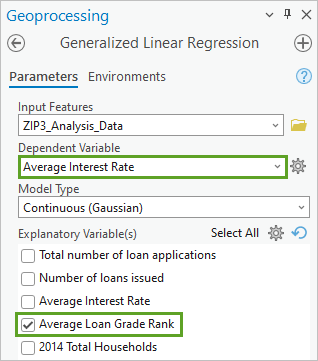The Model Type parameter has three options: Continuous (Gaussian), Binary (Logistic), and Count (Poisson). The option you select is based on the dependent variable. When you looked at the attribute table, you learned that the interest rates were continuous values with decimal places, not binary values or discrete counts.

5. Leave Model Type set to Continuous (Gaussian).

This model type will perform Ordinary Least Squares regression, which provides a global model of the dependent variable and creates a single regression equation to represent it.

6. For Output Features, change the output name to Average_Interest_Rates_vs_Loan_Grades.
7. Click Run.

The tool runs. A layer is added to the map. Three charts are added to the Contents pane.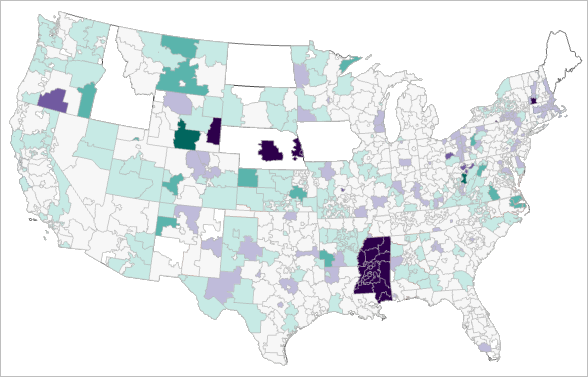This layer maps the residuals of the regression model (where the model's predictions were higher or lower than the actual values). The purple areas are locations where average interest rates were lower than the model predicted, while the green areas are locations where the interest rates were higher.

The spatial pattern of residuals is not random. In particular, the entire state of Mississippi has a large cluster of ZIP3 areas where the model predicted higher interest rates than were observed.

Examine the regression results

Your regression analysis also created a report and several charts. First, you'll examine the report.

1. At the bottom of the Geoprocessing pane, point to View Details.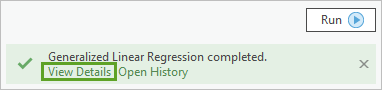The Generalized Linear Regression tool report appears.

2. In the Generalized Linear Regression tool report, scroll down and expand messages to review the GLR Diagnostics.
Tip:

You can resize the tool report by dragging its edges.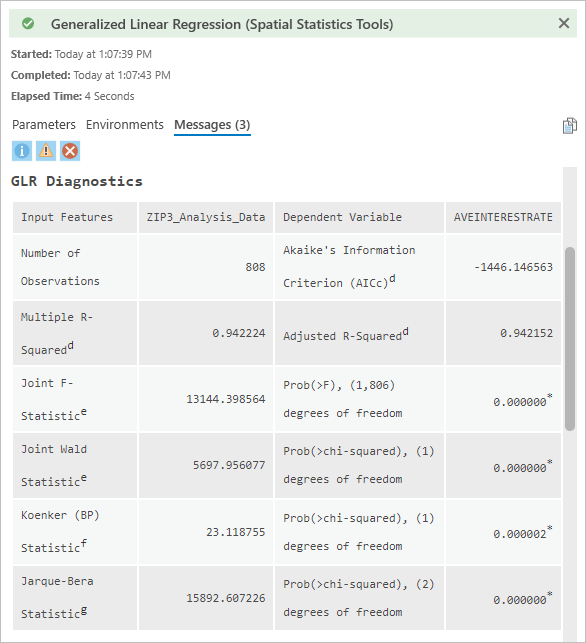For now, you're only interested in the adjusted R-squared value. The R-squared value ranges from 0 to 100 percent (expressed as a decimal) and indicates the strength of the correlation between average interest rates and average loan grade rankings.

Under GLR Diagnostics, the Adjusted R-Squared value is 0.942152.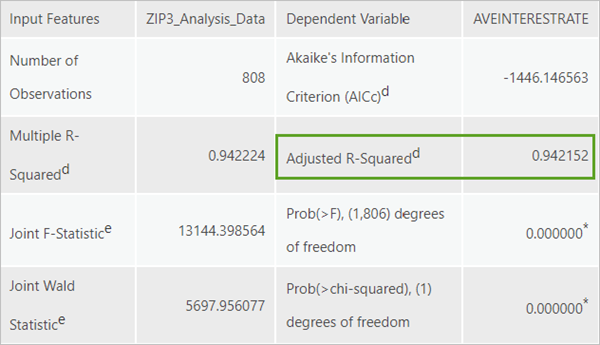This value indicates that the average loan grade rank values explain about 94 percent of the average interest rate values. As expected, this is a high adjusted R-squared value, indicating a strong correlation.

Next, you'll open the scatterplot chart showing the relationship between variables.

3. Close the tool report. In the Contents pane, double-click the Relationship between Variables chart.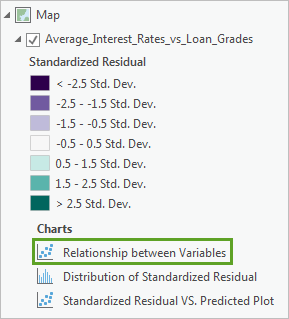The chart appears. The Chart Properties pane also appears.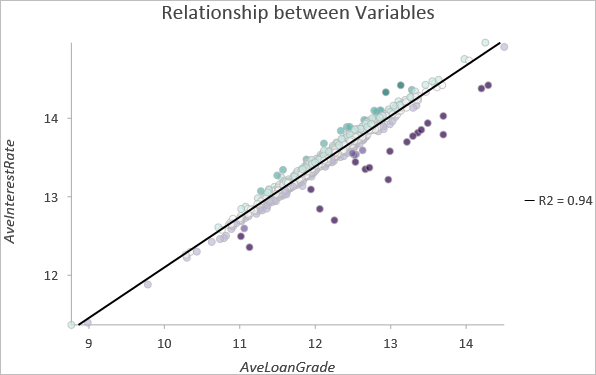The chart plots all ZIP3 areas based on average interest rate and average loan grade. Most of the points follow a straight line, indicating the correlation is strong. The purple points below the line represent ZIP3 areas where the model underpredicted average interest rates.

Although there are several residuals below the line, these still indicate a positive relationship of average interest rate increasing as average loan grade does.

4. Close the chart and the Chart Properties pane. Save the project.

You've used regression analysis to explain average interest rates based on average loan grades. The results were not what Jonathan Blum expected, however. While he did note a strong relationship between average loan grade rankings and average interest rates, he immediately noticed a problem with the residual map. Jonathan expected a random pattern of overpredictions and underpredictions, but there is nothing spatially random about lower-than-expected interest rates for an entire state. Apparently, average loan grade rankings are not an effective predictor of average interest rates in that part of the country.

According to Jonathan, finding lower-than-expected interest rates throughout the state of Mississippi is important. It gives the impression of either intentional bias or disparate impact. Disparate impact can occur when loan decisions that are not intentionally discriminatory result in discriminatory outcomes. A policy of only funding home loans above \$200,000, for example, could have the unintended impact of redlining if the average home values in a region's minority neighborhoods are less than \$200,000. Avoiding disparate impact is difficult for lenders because it isn't exposed until many loans have been made.

Next, you'll use Geographically Weighted Regression to map where the relationship between average loan grades and average interest rates is strong and where it is weak across the country.

Map correlation variations

Previously, you modeled average interest rates as a function of average loan grades. The residual map you created indicated that average loan grades are not good predictors of average interest rates in the state of Mississippi.

When the relationship between two variables is strong, you can predict the value of one from the other. The Generalized Linear Regression (GLR) method you used in the previous lesson summarizes relationship strength using a single coefficient. In other words, it assumes the relationship between average loan grades and average interest rates is the same for every ZIP3 area in the country. If Jonathan Blum wants to examine how this relationship changes, and see where average loan grade rankings have a larger or smaller impact on average interest rates, he needs to learn about another regression technique called Geographically Weighted Regression (GWR).

GWR computes a coefficient for each ZIP3 area. Where coefficients are large, changes in the average loan grade ranking will have a larger impact on average interest rates; where coefficients are small, changes will have a smaller impact.

Next, you'll create a map of the GWR coefficients to identify where the relationship between these two variables is strong and where it is weak.

Find the minimum neighbor distance

GWR calibrates a local regression model for each ZIP3 area using only nearby ZIP3 areas. It also weights nearer features so that they have more influence during calibration than features that are farther away. The Neighborhood Type and Local Weighting Scheme parameters determine which neighboring features are in or out of the calibration process.

For this workflow, you'll try all four combinations of these parameters to see which produces the best results. You can let the tool suggest minimum and maximum search distances and number of neighbors, but the tool will be conservative, requiring a minimum of 30 neighbors. You saw that the relationship between average interest rates and average loan grades is strong, with few outliers. Consequently, your best model will likely use a smaller distance and smaller number of neighbors than the tool might suggest. You'll try distances for between 10 and 50 neighbors.

1. If necessary, open your OnlineLending project.
2. In the Geoprocessing pane, search for and open the Calculate Distance Band from Neighbor Count tool.

You'll use this tool to identify the minimum distance needed for all ZIP3 areas to have at least 10 neighbors.

3. Enter the following parameters:
• For Input Features, choose ZIP3_Analysis_Data.
• For Neighbors, type 10.
• For Distance Method, choose Euclidean.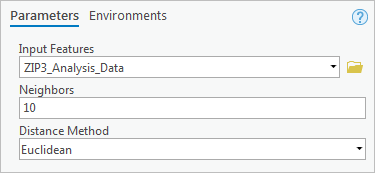4. Click Run.

The tool runs, but no new layers or charts are added to the map or Contents pane.

5. At the bottom of the Geoprocessing pane, click View Details.

The tool report appears. It shows the minimum, average, and maximum distance (in meters) for a ZIP3 area to have at least 10 neighbors. The minimum distance is 17,802 meters, while the maximum is 493,120 meters. The maximum value is the minimum distance needed for every ZIP3 area to have at least 10 neighbors.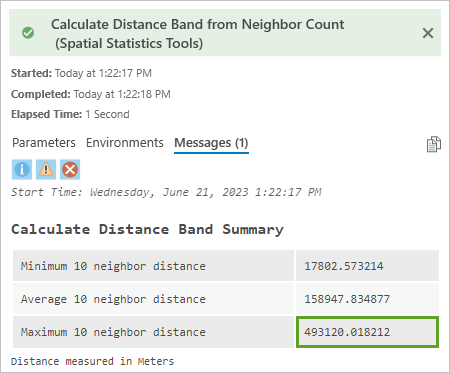You'll round this value down to 400,000 and use it when you perform GWR. Next, you'll make the same calculation to determine the distance needed for a ZIP3 area to have 50 neighbors.

6. Close the tool report. Run the Calculate Distance Band from Neighbor Count tool again, changing the Neighbors parameter to 50.
7. Open the tool report.

The distance needed for every ZIP3 area to have at least 50 neighbors is 1,137,020 meters. You'll round this value down to 1,100,000 and use it when you perform GWR.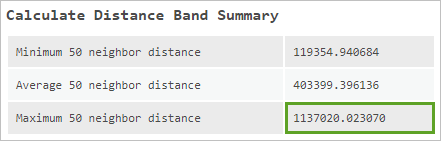8. Close the tool report.

Build the spatial regression model

You'll run the Geographically Weighted Regression (GWR) tool four times with different parameters and map the coefficients for the model that produces the best results.

1. In the Geoprocessing pane, search for and open the Geographically Weighted Regression (GWR) tool. Expand Additional Options.

First, you'll try Number of neighbors for the Neighborhood Type setting. This option uses a fixed number of neighbors for each ZIP3 area, instead of a fixed distance. The Number of neighbors option is generally best when you want to build each local model with the same amount of information. It's a good option when the features are evenly spread out, when the polygons being analyzed are about the same size, or when the underlying spatial processes are homogeneous.

2. Enter the following parameters:
• For Input Features, choose ZIP3_Analysis_Data.
• For Dependent Variable, choose Average Interest Rate.
• For Model Type, choose Continuous (Gaussian).
• For Explanatory Variable(s), choose Average Loan Grade Rank.
• For Output Features, change the output name to GWR_Average_Interest_Rate_vs_Average_Loan_Grade.
• For Neighborhood Type, choose Number of neighbors.
• For Neighborhood Selection Method, choose Manual Intervals.
• For Minimum Number of Neighbors, type 10.
• For Number of Neighbors Increment, type 4.
• For Number of Increments, type 11.
• For Local Weighting Scheme, choose Bisquare.

With these parameters, the tool will run for 10 neighbors, then 14, and then 18, up to 50 neighbors (11 increments of 4). Because of the Bisquare option, features that aren't considered neighbors will have no influence over the results, which could be important for data with strongly localized spatial processes.

3. Click Run.

The tool runs and a report is generated (a layer is also added to the map, but you'll look at it later).

4. Click View Details. Resize the tool report if necessary.

A model was created for each 4-neighbor increment between 10 and 50 neighbors. An adjusted Akaike Information Criterion (AICc) diagnostic was computed for each model. AICc is a value that measures information loss in a model. The lower the AICc, the better the model performs.

The GWR output report's Number of Neighbors value shows the number of neighbors with the lowest AICc. For your report, that number is 22. The AdjR2 (adjusted R-squared) value indicates that this model explains 97.19 percent of variation in the average interest rate values, an improvement over the adjusted R-squared value for your GLR model (94.215 percent).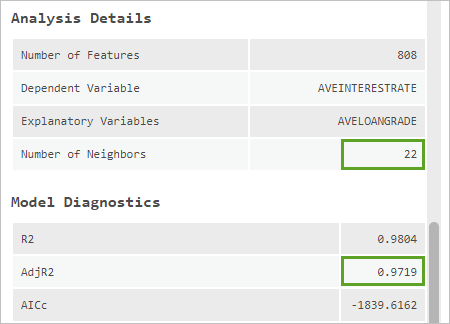Next, you'll run the tool again, with Local Weighting Scheme set to Gaussian. With this setting, all neighboring features (up to the closest 1,000) influence the model, but features beyond the first 10, 14, 18, and so on, have much less influence.

5. Close the tool report. Run the Geographically Weighted Regression (GWR) tool again, changing Local Weighting Scheme to Gaussian.

When you run the tool, the GWR_Average_Interest_Rate_vs_Average_Loan_Grade layer is overwritten with the new results.

6. Click View Details.

With the Gaussian weighting scheme, the best-performing model has 10 local neighbors. However, the AICc value (-1673.8710) is not as small as for the model with 22 neighbors and the Bisquare weighting scheme (-1839.6162). Also, the adjusted R-squared value (0.9594) is smaller than the one produced by the Bisquare option (0.9719).

While better than GLR, the model does not predict as well as the previous GWR model. Next, you'll run the tool again. Instead of using a specific number of neighbors, you'll use the minimum neighbor distances you calculated in the previous sections. For each ZIP3 area to have 10 neighbors, you determined a distance of 400,000 meters was necessary. For each ZIP3 area to have 50 neighbors, the distance needed is 1,100,000 meters.

The Distance band option for Neighborhood Type means neighboring features within the specified distance are used to calibrate each local model. This option has the advantage of ensuring the scale of analysis remains constant. It is most appropriate when you're confident each feature will have sufficient neighbors within the specified distance band to create a reliable local model.

7. Close the tool report. For the Geographically Weighted Regression (GWR) tool, change the following parameters:
• Change Neighborhood Type to Distance band.
• Set Minimum Search Distance to 400000 Meters.
• Set Search Distance Increment to 100000 Meters.
• Set Number of Increments to 8.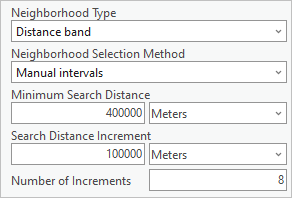With these parameters, the tool will create models for each 100,000-meter interval between 400,000 and 1,100,000 meters.

8. Run the tool. When the tool finishes, click View Details.

The best-performing distance band is 400,000 meters, but the result is still not as good as the first GWR model you tried (its AICc is -1565.1312 and its adjusted R-squared value is 0.9507).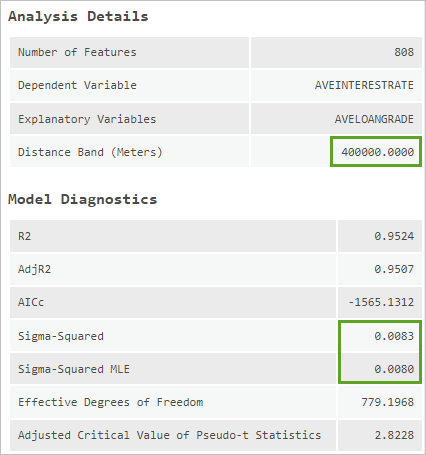You'll run the model one more time. You'll use the same distance band parameters, but change the local weighting scheme.

9. Close the tool report. Run the Geographically Weighted Regression (GWR) tool again, changing Local Weighting Scheme to Bisquare.
10. Open the report.

This model performs better than the previous one, but it is still not as effective as the first model you tried. While this model's AICc (-1843.3228) is slightly smaller than the first model you tried (-1839.6162), its adjusted R-squared value is also smaller (0.9676 compared to 0.9719).

You've identified the model parameters producing the smallest AICc value in conjunction with the largest adjusted R-squared value. These diagnostics indicate that performing GWR with a fixed number of 22 neighbors and a Bisquare weighting scheme produces the best-performing model. You can use a similar workflow to compare any models that have the same dependent variable.

Every time you ran the model, you overwrote the previous model's results. You'll run the model with the same parameters as the first time you ran it in order to re-create the best result output.

11. Close the tool report. Run the tool with Neighborhood Type set to Number of Neighbors, Neighborhood Selection Method set to User defined, and Number of Neighbors set to 22.
12. Save the project.

Map the model coefficients

You've identified the model parameters that produce the smallest AICc value in conjunction with the largest adjusted R-squared value, indicating the best model. Next, you'll map the model coefficients to examine how the relationship between average interest rates and average loan grades changes across the country.

Like the map output from GLR, the map output from GWR shows the residuals (where the model predictions are either higher or lower than the actual average interest rate values). The output layer also contains a field with the coefficient value for each ZIP3 area. The larger the coefficient, the stronger the relationship is between average interest rates and average loan grades. Mapping this field will provide insight into the relationship between these variables across the country.

1. In the Contents pane, right-click the GWR_Average_Interest_Rate_vs_Average_Loan_Grade layer and choose Symbology.

The layer's Symbology pane appears.

Note:

You may need to change Primary symbology to Unique Values and change it back to Graduated Colors for the new symbology to display.

2. Set Field to Coefficient (AVELOANGRADE), Method to Quantile, and Classes to 7.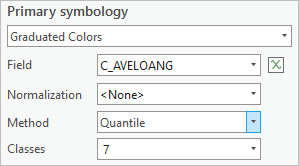3. For Color scheme, choose the Yellow-Orange-Brown continuous color ramp (or any graduated color ramp that represents data arranged from smallest to largest).
Tip:

To view the name of a color scheme, point to it.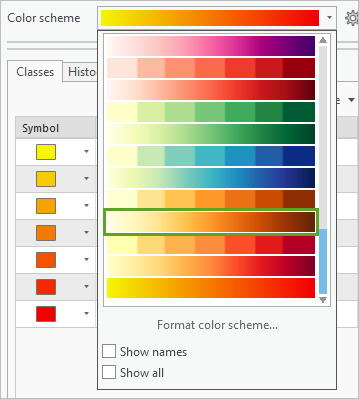4. Close the Symbology pane. In the Contents pane, drag the State Boundaries layer above the GWR_Average_Interest_Rate_vs_Average_Loan_Grade layer.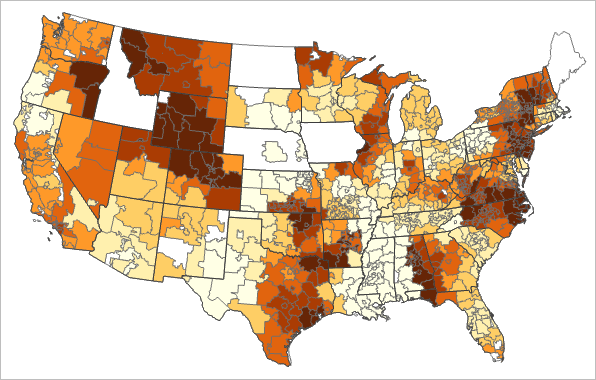On the map, darker areas are places where the relationship between the two variables is strong. Lighter areas are places where the relationship is weak.

5. Save the project.

The map suggests that interest rates are not solely dependent on loan grades, at least not everywhere. In both Mississippi and much of Kansas, for example, there is a weak relationship between average loan grades and average interest rates. Interest rates are lower than expected, on average, throughout Mississippi. They are higher than expected, however, in much of Kansas.

This pattern has tangible and material consequences. Differences in loan interest rates impact the entire economy. When access to loans is limited because of high interest rates, people tend to spend less and businesses tend to downscale. When loan interest rates are low, people are more willing to borrow and spend, and businesses are more likely to expand.

Some researchers have found evidence of discrimination in a variety of online marketplaces. Jonathan Blum's exploratory analysis contributes to this research area by uncovering evidence of geographic discrimination associated with online lending. Jonathan has only considered loan grades, however. Despite LendingClub indicating a direct relationship between loan grades and interest rates, the maps you created suggest other factors are involved. For example, some researchers have found that as many as one-third of borrowers will choose the loan with the fastest funding time over the one with the lowest interest rate.

Jonathan is a journalist. His job is to report and inform emerging debates around online lending. The maps created and analyses performed in this lesson are critical storytelling tools that he will be able to use broadly in his work.

In this lesson, you used spatial regression analysis to model the relationship between average interest rates and average loan grade rankings, testing an assumed correlation. You can use this workflow to test other assumed correlations. Communities with higher average incomes, for example, will likely pay higher average income taxes. But is this consistently true? Where is it less true or more consistent across the country? Agricultural areas with the best growing conditions should produce the highest yields. Is that the case everywhere? If not, why not? Wouldn't it be reasonable to assume schools with better teacher-to-student ratios have higher test scores?

What are you waiting for? Start testing some of your own assumed relationships and see what you discover.

You can find more lessons in the Learn ArcGIS Lesson Gallery.